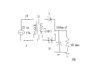# Filter circuit

• Engineering
pooface
Hello I'm a beginner (Second Semester) at Electronics Engineering.

In the attachment is the image of the filter rectifier circuit that I am analyzing. My professor has given us questions and their solutions but for my full wave filter rectifier analysis the numbers are not the same.

The analysis asks me three questions:

VDC, Vr, and ripple %

Formulas I use:

Vrp-p=(VLpeak/RL * period) / C (capacitor value).

VDC = VLpeak - Vrpeaktopeak/2

Vr(rms)=.577* Vrp-p/2

r=Vr(rms)/VDC

My halfwave filter answers are correct and these are the formulas I used.
But my fullwave/bridge answers go wrong. someone please do this question and see what answer they get.

#### Attachments

•rfc.JPG
12.3 KB · Views: 420
Last edited:

Gold Member
A half wave and full wave rectifier differ in certain ways. You can't apply all formulas (or same value for period) to both. What value are you using for period, T?

Vrpp = (Vpin * T)/RC

Please so your exact calculations, like for example how you got Vrrms

Last edited:
triden
Have to remember, in a half wave rectifier the frequency is 60 hz as it chops off the bottom half of each cycle. In a full wave circuit, it translates the bottom cycle to the top to give 120hz peaks.

pooface
A half wave and full wave rectifier differ in certain ways. You can't apply all formulas (or same value for period) to both. What value are you using for period, T?

Vrpp = (Vpin * T)/RC

Please so your exact calculations, like for example how you got Vrrms
EDIT:

Nevermind thanks so much ! i got it. You were right. I was using the wrong T value. Fullwave is 120Hz so i should be using 1/120hz for T.

The numbers add up now. Thanks so much.

Last edited: